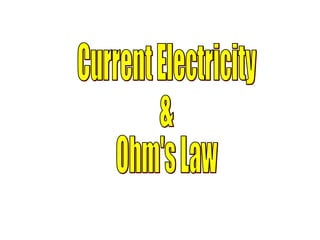Diese Präsentation wurde erfolgreich gemeldet.

# Current Electricity & Ohms Law

Anzeige
Anzeige
Anzeige
Anzeige
Anzeige
Anzeige
Anzeige
Anzeige
Anzeige
Anzeige
Anzeige×

1 von 22 Anzeige

# Current Electricity & Ohms Law

Discusses Ohm's Law and current electricity and related to energy transfer in circuits.
**More good stuff available at:
www.wsautter.com
and

Discusses Ohm's Law and current electricity and related to energy transfer in circuits.
**More good stuff available at:
www.wsautter.com
and

Anzeige
Anzeige

### Current Electricity & Ohms Law

1. 1. Current Electricity & Ohm's Law
2. 2. The next slide is a quick promo for my books after which the presentation will begin Thanks for your patience! Walt S. [email_address] More stuff at: www. wsautter .com
4. 4. <ul><li>A source voltage, that is, an electron pump usually </li></ul><ul><li>a battery or power supply. </li></ul><ul><li>[ ENERGY IN] </li></ul>Circuit Basics (2) A conductor to carry electrons from and to the voltage source (pump). The conductor is often a wire. [ENERGY TRANSFER] (3) A load or resistance. A point where energy is extracted form the circuit in the form of heat, light, motion, etc. [ENERGY OUT] All electrical circuits require three elements.
5. 5. ELECTRON PUMP (SOURCE VOLTAGE) [ENERGY IN] LOAD (RESISTANCE) [ENERGY OUT] CONDUCTOR ELECTRONS OUT OF SOURCE ELECTRONS OUT OF LOAD ELECTRONS BACK TO SOURCE ELECTRONS INTO LOAD HIGHER ENERGY ELECTRONS LOWER ENERGY ELECTRONS THE MOST BASIC ELECTRICAL CIRCUIT CONDUCTOR
6. 6. Voltage Source (Potential Rise) Low Energy current High Energy current Resistance (Potential Drop) Low Energy current High Energy current Potential Changes of Current in a Circuit
7. 7. Battery current Potential Rise Across a Power Source Electrons get An energy boost current volts Electrons have Less Energy Electrons have More Energy
8. 8. Resistor current Potential Drop Across a Resistor Energy is lost In the resistor volts Electrons have More Energy Electrons have Less Energy
9. 9. MEASUREABLE QUANTITIES THAT CAN BE OBTAINED FROM AN ELECTRICAL CIRCUIT (1) VOLTAGE RISE – MEASURES THE ENERGY GIVEN TO ELECTRONS AS THEY LEAVE A VOLTAGE SOURCE. IT IS MEASURED IN VOLTS (+) (2) VOLTAGE DROP – MEASURES THE ENERGY LOST BY TO ELECTRONS WHEN THEY LEAVE A RESISTANCE. IT IS MEASURED IN VOLTS (-) (3) CURRENT – MEASURES THE FLOW RATE THROUGH A CONDUCTOR. IT IS MEASURED IN AMPERES (AMPS) (4)RESISTANCE – MEASURES THE OPPOSITION TO CURRENT FLOW THROUGH A CONDUCTOR OR RESISTOR IT IS MEASURED IN OHMS (ITS SYMBOL IS OMEGA )
10. 10. 6.25 x 10 18 electrons 1 coulomb 1 coulomb 1 second 1 amp = 1 coul / sec 1 joule 1 coulomb of charge 1 volt = 1 joule / coul ELECTRICAL QUANTITIES
11. 11. AMPS volts Ammeters measure current in amperes and are always wired in series in the circuit. Voltmeters measure potential in volts and are always wired in parallel in the circuit. Electrical Meters
12. 12. An actual Voltmeter
13. 13. An Actual Ammeter
14. 14. Electrical Symbols wiring battery voltmeter ammeter resistance capacitor junction terminal AC generator Variable resistance Variable capacitor + - A V
15. 15. Relationships Among Electrical Quantities in a Circuit Measure electrical quantities in an electrical Circuit are related to eachother by: OHM’S LAW OHM’S LAW says, if the source voltage remains constant, increasing the resistance in a circuit will cause a decrease in current flow in that circuit. In mathematical terms it tells us that current flow is inversely proportional to resistance. In equation form it says: Voltage (V) = Current (I) x Resistance (R) or V(in volts) = I (in amps) x R (in ohms)
16. 16. V = I R Ohm's Law Drop across a resistance Current passing Through the resistor Potential In volts (joules / coul) Current In amperes (coul / second) Resistance In ohms (volts / amp)
17. 17. Voltage vs Current for a Constant Resistance The slope of the Line gives the resistance R =  v /  I or rearranged  v =  I x R V O L T A G E (volts) Current (I) in amps  I  v
18. 18. Voltage Sources and Internal Resistance All voltage sources contain internal resistance , that is resistance that is part of the voltage producing device itself which cannot be eliminated. The voltage that the device (battery for example) could produce if no internal resistance was present is called its EMF. EMF stands for electromotive force – the force that moves the electrons . The useable voltage which is available to the circuit after the internal resistance consumes its share of the EMF is called The terminal voltage.
19. 19. Electromotive Force (EMF) and Terminal Voltage conventional current flow EMF Voltage rise Voltage drop EMF of Source Internal resistance + - Terminal voltage
20. 20. terminal voltage = EMF (+) + Internal resistance loss (-) Since voltage rise across a source or Voltage drop across a resistance can be Calculated by Ohm’s Law V= IR And the above equation becomes V terminal = EMF – I x R internal Note that if R interna l is very small then a large Percentage of the EMF is available to the circuit. Also note that if I, the current is very large then a large percentage of the EMF is consumed within the battery itself which can cause overheating and failure. This is generally the result of a short circuit.
21. 21. Battery current Internal Resistance and EMF current EMF R i volts Voltage rise Due to EMF Voltage drop Due to internal resistance +V -V Net Voltage Rise of cell (Terminal Voltage
22. 22. THE END